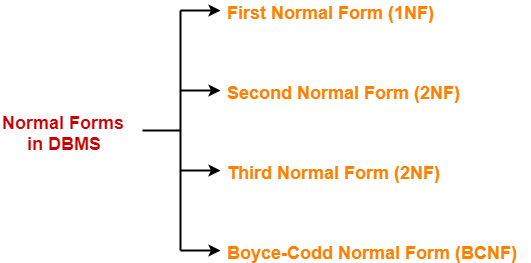# Normalization in DBMS | Normal Forms

## Normalization in DBMS-

In DBMS, database normalization is a process of making the database consistent by-

• Reducing the redundancies
• Ensuring the integrity of data through lossless decomposition

Normalization is done through normal forms.

## Normal Forms-

The standard normal forms used are-1. First Normal Form (1NF)
2. Second Normal Form (2NF)
3. Third Normal Form (3NF)
4. Boyce-Codd Normal Form (BCNF)

There exists several other normal forms even after BCNF but generally we normalize till BCNF only.

## First Normal Form-

A given relation is called in First Normal Form (1NF) if each cell of the table contains only an atomic value.

OR

A given relation is called in First Normal Form (1NF) if the attribute of every tuple is either single valued or a null value.

### Example-

The following relation is not in 1NF-

 Student_id Name Subjects 100 Akshay Computer Networks, Designing 101 Aman Database Management System 102 Anjali Automata, Compiler Design

Relation is not in 1NF

However,

• This relation can be brought into 1NF.
• This can be done by rewriting the relation such that each cell of the table contains only one value.

 Student_id Name Subjects 100 Akshay Computer Networks 100 Akshay Designing 101 Aman Database Management System 102 Anjali Automata 102 Anjali Compiler Design

Relation is in 1NF

This relation is in First Normal Form (1NF).

### NOTE-

• By default, every relation is in 1NF.
• This is because formal definition of a relation states that value of all the attributes must be atomic.

Second Normal Form-

A given relation is called in Second Normal Form (2NF) if and only if-

1. Relation already exists in 1NF.
2. No partial dependency exists in the relation.

Also Read- Functional Dependency in DBMS

### Partial Dependency

A partial dependency is a dependency where few attributes of the candidate key determines non-prime attribute(s).

OR

A partial dependency is a dependency where a portion of the candidate key or incomplete candidate key determines non-prime attribute(s).

In other words,

A → B is called a partial dependency if and only if-

1. A is a subset of some candidate key
2. B is a non-prime attribute.

If any one condition fails, then it will not be a partial dependency.

### NOTE-

• To avoid partial dependency, incomplete candidate key must not determine any non-prime attribute.
• However, incomplete candidate key can determine prime attributes.

Also Read- How To Find Candidate Keys?

## Example-

Consider a relation- R ( V , W , X , Y , Z ) with functional dependencies-

VW → XY

Y → V

WX → YZ

The possible candidate keys for this relation are-

VW , WX , WY

From here,

• Prime attributes = { V , W , X , Y }
• Non-prime attributes = { Z }

Now, if we observe the given dependencies-

• There is no partial dependency.
• This is because there exists no dependency where incomplete candidate key determines any non-prime attribute.

Thus, we conclude that the given relation is in 2NF.

Third Normal Form-

A given relation is called in Third Normal Form (3NF) if and only if-

1. Relation already exists in 2NF.
2. No transitive dependency exists for non-prime attributes.

### Transitive Dependency

A → B is called a transitive dependency if and only if-

1. A is not a super key.
2. B is a non-prime attribute.

If any one condition fails, then it is not a transitive dependency.

### NOTE-

• Transitive dependency must not exist for non-prime attributes.
• However, transitive dependency can exist for prime attributes.

OR

A relation is called in Third Normal Form (3NF) if and only if-

Any one condition holds for each non-trivial functional dependency A → B

1. A is a super key
2. B is a prime attribute

## Example-

Consider a relation- R ( A , B , C , D , E ) with functional dependencies-

A → BC

CD → E

B → D

E → A

The possible candidate keys for this relation are-

A , E , CD , BC

From here,

• Prime attributes = { A , B , C , D , E }
• There are no non-prime attributes

Now,

• It is clear that there are no non-prime attributes in the relation.
• In other words, all the attributes of relation are prime attributes.
• Thus, all the attributes on RHS of each functional dependency are prime attributes.

Thus, we conclude that the given relation is in 3NF.

## Boyce-Codd Normal Form-

A given relation is called in BCNF if and only if-

1. Relation already exists in 3NF.
2. For each non-trivial functional dependency A → B, A is a super key of the relation.

## Example-

Consider a relation- R ( A , B , C ) with the functional dependencies-

A → B

B → C

C → A

The possible candidate keys for this relation are-

A , B , C

Now, we can observe that RHS of each given functional dependency is a candidate key.

Thus, we conclude that the given relation is in BCNF.

Next Article- Important Points About Normal Forms

Get more notes and other study material of Database Management System (DBMS).

Watch video lectures by visiting our YouTube channel LearnVidFun.

SummaryArticle Name
Normalization in DBMS | Normal Forms
Description
Normalization in DBMS is a process of making database consistent. Normal Forms in DBMS- First Normal Form (1NF), Second Normal Form (2NF), Third Normal Form (3NF), Boyce Codd Normal Form (BCNF).
Author
Publisher Name
Gate Vidyalay
Publisher Logo